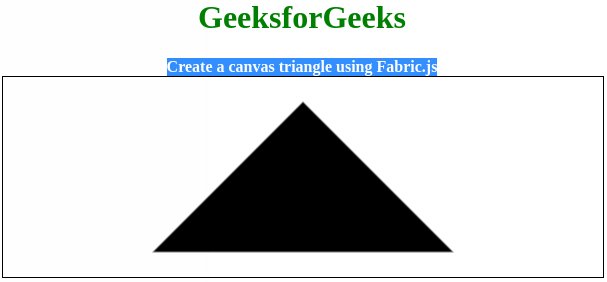# How to create a canvas triangle using Fabric.js ?

• Last Updated : 29 May, 2020

In this article, we are going to create a canvas triangle using FabricJS. The canvas triangle means the triangle is movable and can be stretched according to requirement. Further, the triangle can be customized when it comes to initial stroke color, height, width, fill color, or stroke width.

To make it possible we are going to use a JavaScript library called FabricJS. After importing the library, we will create a canvas block in the body tag that will contain the triangle. After this, we will initialize instances of Canvas and Triangle provided by FabricJS and render the Triangle instance on the Canvas instance.

Syntax:

```fabric.Triangle({
width: number,
height: number,
});```

Parameters: This function accepts two parameters as mentioned above and described below:

• width: It specifies the width of triangle.
• height: It specifies the height of triangle.

Example: This example uses FabricJS to create simple editable canvas triangle.

 ` `` `` ` ` ``    `` ``        ``How to create a canvas triangle using Fabric.js ?``    `` ``     ` `    ````    `` `` `` ` ` ``    ``
``        ``

``          ``GeeksforGeeks ``        ``

``        `` ``              ``Create a canvas triangle using Fabric.js  ``        `` ``    ``
``    `` ``    `` ``     ` `    `` `` `` ` ``

Output:My Personal Notes arrow_drop_up